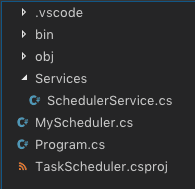Hello Guys, Today I’m going to create a very simple Task Scheduler using c# without using any Library. Using this Task Scheduler we’ll be able to Schedule a Task by Seconds, Minutes, Hours and Days.

A Few Days ago, I was working on one of my projects & met with a challenge where I needed to run a task every 30 minutes and another task daily at 2 am. After searching a bit I found answers about Quartz.net. But it seems to be too big for my project. I want an equivalent but the simpler solution for my Project. So I decided to create my own Task Scheduler.

In this article, I’m going to show you how you can create a very Simple Task Scheduler using very clean code.

Let’s start by creating a console project.

Follow the best practice & create a new folder with the name as “Services” & inside this Folder create a new File as “SchedulerService.cs”

Now paste this code in the newly created File.

```using System;
using System.Collections.Generic;

public class SchedulerService
{
private static SchedulerService _instance;
private List<Timer> timers = new List<Timer>();

private SchedulerService() { }

public static SchedulerService Instance => _instance ?? (_instance = new SchedulerService());

{
DateTime now = DateTime.Now;
DateTime firstRun = new DateTime(now.Year, now.Month, now.Day, hour, min, 0, 0);
if (now > firstRun)
{
}

TimeSpan timeToGo = firstRun - now;
if (timeToGo <= TimeSpan.Zero)
{
timeToGo = TimeSpan.Zero;
}

var timer = new Timer(x =>
{
}, null, timeToGo, TimeSpan.FromHours(intervalInHour));

}
}```

Now create another File inside your root directory as “MyScheduler.cs” and paste this code

```using System;

public static class MyScheduler
{
public static void IntervalInSeconds(int hour, int sec, double interval, Action task)
{
interval = interval/3600;
}

public static void IntervalInMinutes(int hour, int min, double interval, Action task)
{
interval = interval/60;
}

public static void IntervalInHours(int hour, int min, double interval, Action task)
{
}

public static void IntervalInDays(int hour, int min, double interval, Action task)
{
interval = interval * 24;
}
}```

Now you have your project structure something like this.Now It’s time to use the scheduler from your “Program.cs”

Running a Scheduler is very simple you have four methods => “IntervalInSeconds”, “IntervalInMinutes”, “IntervalInHours” & “IntervalInDays”, you just need to call one of these methods & pass the first 2 parameters as starting hour & minutes. If specified start time has over scheduling start on the next day at that time.

So here’s the Code for “Program.cs”

```using System;

{
class Program
{
static void Main(string[] args)
{
// For Interval in Seconds
// This Scheduler will start at 11:10 and call after every 15 Seconds
// IntervalInSeconds(start_hour, start_minute, seconds)
MyScheduler.IntervalInSeconds(11, 10, 15,
() => {

Console.WriteLine("//here write the code that you want to schedule");
});

// For Interval in Minutes
// This Scheduler will start at 22:00 and call after every 30 Minutes
// IntervalInSeconds(start_hour, start_minute, minutes)
MyScheduler.IntervalInMinutes(22, 00, 30,
() => {

Console.WriteLine("//here write the code that you want to schedule");
});

// For Interval in Hours
// This Scheduler will start at 9:44 and call after every 1 Hour
// IntervalInSeconds(start_hour, start_minute, hours)
MyScheduler.IntervalInHours(9, 44, 1,
() => {

Console.WriteLine("//here write the code that you want to schedule");
});

// For Interval in Seconds
// This Scheduler will start at 17:22 and call after every 3 Days
// IntervalInSeconds(start_hour, start_minute, days)
MyScheduler.IntervalInDays(17, 22, 3,
() => {

Console.WriteLine("//here write the code that you want to schedule");
});

}
}
}
```

Comment If you find any problem. You can also download complete code for this Scheduler from Github.

## Limitations

One of our Community member Umar Tarar found a very valid point…

Using System.Threading.Timer has a limitation on its ‘period’ param i.e, the maximum interval that you can set for the timer is 49 days 17 hours 2 min 47 sec and 294 ms, this is because the max value that can be passed as period (or interval) is the max number that a uint can hold and that is UInt32.MaxValue = 4294967294 in milliseconds. This is because the internal implementation of this timer can accept the interval as uint only even though the wrapper Timer constructor accepts it int, long, Timespan etc.
This limitation is mentioned in the official documentation: Here

Here are more Articles you might be InterestedI'm passionate about learning new technologies as well as mentoring and helping others get started with their programming career. This blog is my way of giving back to the Community.

1.2.